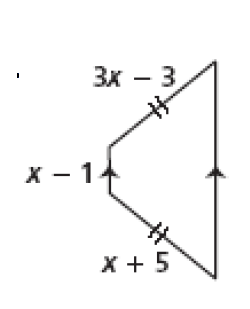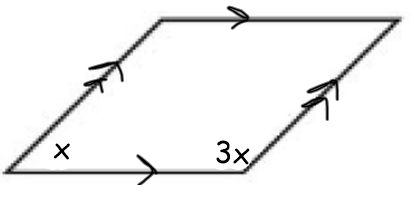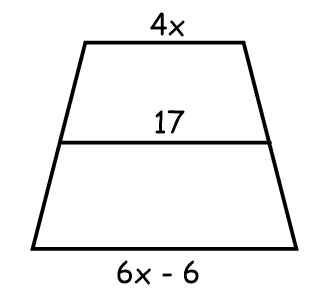*
1 point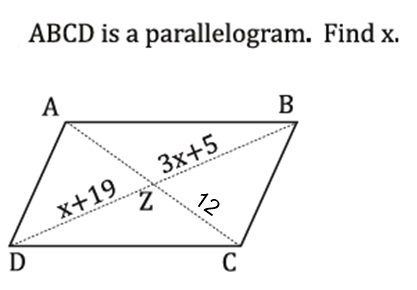Find x if the PERIMETER IS 105 *
1 point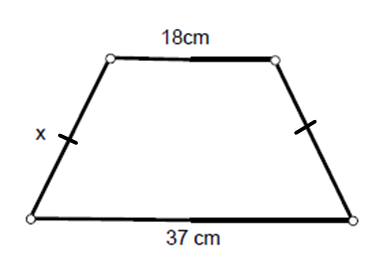find x on this isosceles trapezoid *
1 pointFind x on this parallelogram *
1 point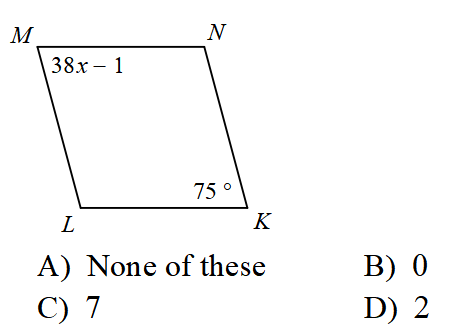find angle y in this rhombus *
1 point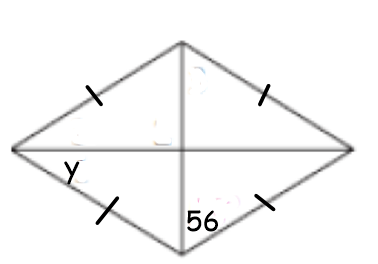FInd angle x in rhombus x *
1 point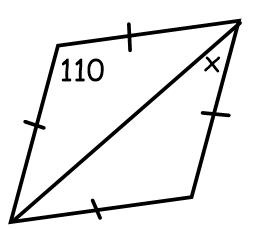Solve for x *
1 point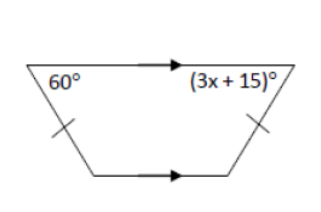Last Name *
Find x on this isosceles trapezoid *
1 point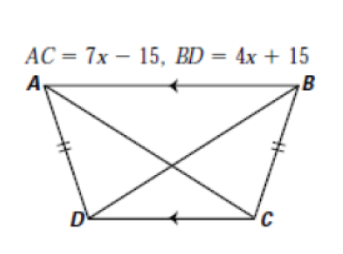Find length of segment EB
1 point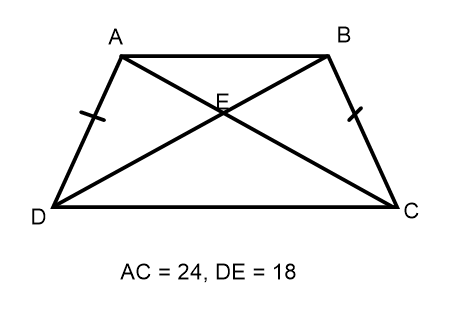find x on this isosceles trapezoid *
1 point